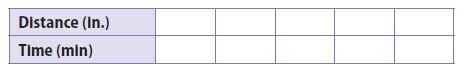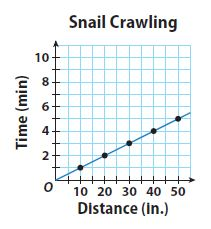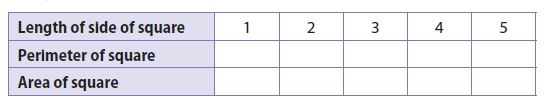Homework Explained - Math Practice 101Dear guest, you are not a registered member. As a guest, you only have read-only access to our books, tests and other practice materials.

As a registered member you can:

Registration is free and doesn't require any type of payment information. Click here to Register.
Go to page:
Chapter 3: Proportional Relationships; Lesson 1: Representing Proportional Relationships

• Question 13

The graph shows the relationship between the distance that a snail crawls and the time that it crawls.
a. Use the points on the graph to make a table.• Type below:
• Question 13

b. Write the equation for the relationship and tell what each variable represents.

• Type below:
• Question 13

c. How long does it take the snail to crawl 85 inches?

•  minutes

H.O.T.

Focus on Higher Order Thinking

• Question 14

Communicate Mathematical Ideas

Explain why all of the graphs in this lesson show the first quadrant but omit the other three quadrants.

• Type below:
• Question 15

Analyze Relationships

Complete the table.a. Are the length of a side of a square and the perimeter of the square related proportionally? Why or why not?

• Question 15

b. Are the length of a side of a square and the area of the square related proportionally? Why or why not?

• Question 16

Make a Conjecture

A table shows a proportional relationship where k is the constant of proportionality. The rows are then switched. How does the new constant of proportionality relate to the original one?

• Type below:

Yes, email page to my online tutor. (if you didn't add a tutor yet, you can add one here)